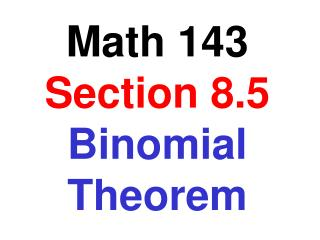Download PresentationMath 143 Section 8.5 Binomial Theorem

# Math 143 Section 8.5 Binomial Theorem - PowerPoint PPT PresentationDownload Presentation## Math 143 Section 8.5 Binomial Theorem

- - - - - - - - - - - - - - - - - - - - - - - - - - - E N D - - - - - - - - - - - - - - - - - - - - - - - - - - -
##### Presentation Transcript

1. Math 143 Section 8.5Binomial Theorem

2. Binomial Expansions (a + b)1 = a + b (a + b)2 = a2 + 2ab + b2 (a + b)3 = a3 + 3a2b + 3ab2 + b3 (a + b)4 = a4 + 4a3b + 6a2b2 + 4ab3 + b4 (a + b)5 = a5 + 5a4b + 10a3b2 + 10a2b3 + 5ab4 + b5 Pasqual's Triangle 1 1 1 2 1 1 3 3 1 1 4 6 4 1 1 5 10 10 5 1

3. Factorial Expressions 5! = 5 · 4 · 3 · 2 · 1 = 120 9! = 9 · 8 · 7 · 6 · 5 · 4 · 3 · 2 · 1 = 362,880 For a non-negative number, n, n! = (n)(n – 1)(n – 2)·. . . 2 · 1 1! = 1 0! = 1

4. We can use Pascal’s triangle to expand binomials, but it becomes large and cumbersome when the powers of the binomial are large. Therefore, the coefficients in a binomial expansion are often given in terms of factorials. Binomial Coefficients n r For nonnegative integers n and r, with n ³r, the expression is called a binomial coefficient and is defined by n r n! = r! (n – r)! 6 2 6! 6 · 5 · 4! 30 = = = = 15 2! · 4! 2! · 4! 2 3! 3 0 = = 1 0! · 3!

5. The Binomial Theorem For any positive integer, n n 0 n 1 n 2 n 3 n n (a + b)n = an + an-1b + an-2b2 + an-3b3 + . . . + bn Expand: (x + 2)5 5 4 5 5 5 0 5 1 5 2 5 3 x5 + x4(2)1 + x3(2)2 + x2(2)3+ x(2)4 + (2)5 = = (1)(x5) + 5(x4)(2) + 10(x3)(4) + 10(x2)(8) + 5(x)(16) + (1)(32) = x5 + 10x4 + 40x3 + 80x2 + 80x + 32

6. Expand: (3x – 2y)4 4 0 4 1 4 2 4 3 4 4 (3x)4 + (3x)3(-2y)1 + (3x)2(-2y)2 + (3x)1(-2y)3+ (-2y)4 = = (1)(81x4) + 4(27x3)(-2y) + 6(9x2)(4y2) + 4(3x)(-8y3) + (1)(16y4) = 81x4 – 216x3y + 216x2y2 – 96xy3 + 16y4 Find the first three terms of the following expansion 20 0 20 1 20 2 (x2 – 1)20 (x2)20 + (x2)19(-1)1 + (x2)18(-1)2 + . . . = = (1)(x40) + 20(x38)(-1) + 190(x36)(1) + . . . = x40 – 20x38+ 190x36 – . . .

7. Find the 8th term of the expansion of (2x – 3)12 12 7 8th term = (2x)5(-3)7 = 792(32x5)(-2187) = - 55,427,328 x5 In the expansion of (x + 2y)15 , find the term containing y8 15 8 the term containing y8 = (x)7(2y)8 = 6435(x7)(256y8) = 1,647,360 x7y8

8. find and simplify f(x + h) – f(x) h f(x) = x5 (x + h)5 – x5 f(x + h) – f(x) h = h = (x5 + 5x4h + 10x3h2 + 10x2h3 + 5xh4 + h5) – x5 h = 5x4h + 10x3h2 + 10x2h3 + 5xh4 + h5 h = 5x4 + 10x3h + 10x2h2 + 5xh3 + h4